Smartick is a fun way to learn math!Apr03

# Learn and Practice How to Divide Decimals

Do you know how to divide decimals? In this post, you will learn how, and at the end, you will find exercises to practice division of decimals.

But first you can review what decimal numbers are with this previous post from our blog: Learn What Decimal Numbers Are.

• ### How to divide a whole number by a whole number with a decimal answer: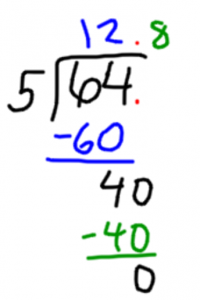When we do division and are left with a remainder, we can continue dividing to get the answer as a decimal. How do we do it? Add a zero to the remainder and a decimal point in the quotient. Then we can continue to divide decimals.

We are going to see it with an example: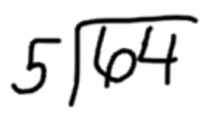We divide 64 by 5 and obtain 12 as a quotient and 4 as a remainder.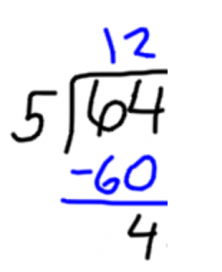Since the remainder is not zero, we can continue to get a decimal answer by adding a decimal point in the quotient and a zero to the remainder.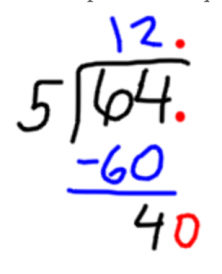Now, we divide 40 by 5. The answer is 8 and the remainder is zero. So we do not have to do any more with the decimals.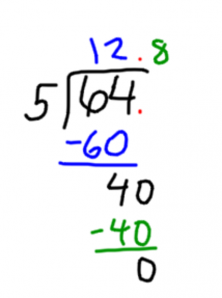• ### How to divide decimals by a whole number:

Divide the same way as a whole number by a whole number, but when we bring down the first decimal digit from the dividend, you have to write a decimal point in the quotient.

Let’s look at an example: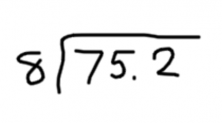We begin dividing 75 by 8, leaving us with 9 as a quotient and 3 as a remainder.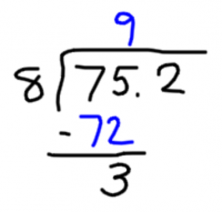Now, we have to bring down the next digit, but be careful! The next digit is the first decimal digit, so we must add a decimal point in the quotient.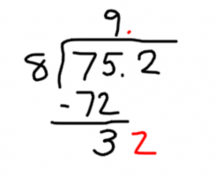Now, we are only left to divide 32 by 8, leaving us with a quotient of 4 and a remainder of zero. Therefore, we have finished doing the division problem.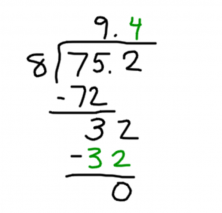• ### How to divide a whole number by a decimal:

You have to get rid of the decimal from the divisor. To do that, we add as many zeros to the dividend as the number of decimal digits in the divisor.

Let’s look at an example: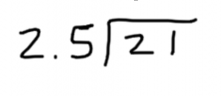In this case, the divisor has one decimal digit. Therefore, we have to add a zero to the dividend and get rid of the decimal point of the divisor.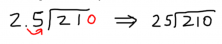Now, we can divide 210 by 25, which gives us 8 with a remainder of 10.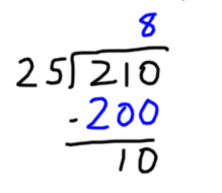Since there is a remainder we continue by adding a decimal point to the quotient and writing a zero in the remainder.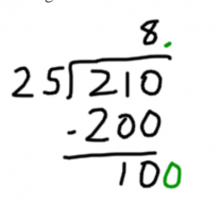Dividing 100 by 25, we obtain the quotient of 4 and a remainder of zero. So we are finished.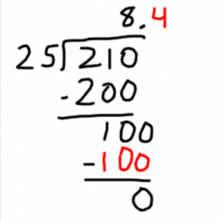• ### How to divide decimals by a decimal:

You have to get rid of the decimal point of the divisor by moving the decimal point of the dividend as many positions to the right as the divisor has decimal digits.

We are going to see it with an example: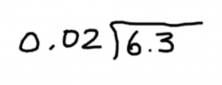In this case, the divisor has 2 decimal digits. Therefore, we move the decimal point of the dividend 2 positions to the right.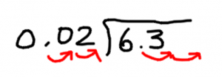Since the dividend only has 1 decimal digit, we fill the empty place with a 0.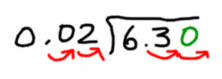Now, we are left with a whole number division problem: 630 by 2, and the answer is 315.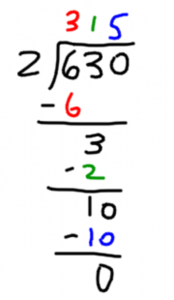If you feel confident with dividing decimals, check out how to add and multiply decimal numbers:

If you liked the post, share it with your friends so they can also learn to do division with decimals! If you want to practice these kinds of problems, and more, go to Smartick and try it for free.